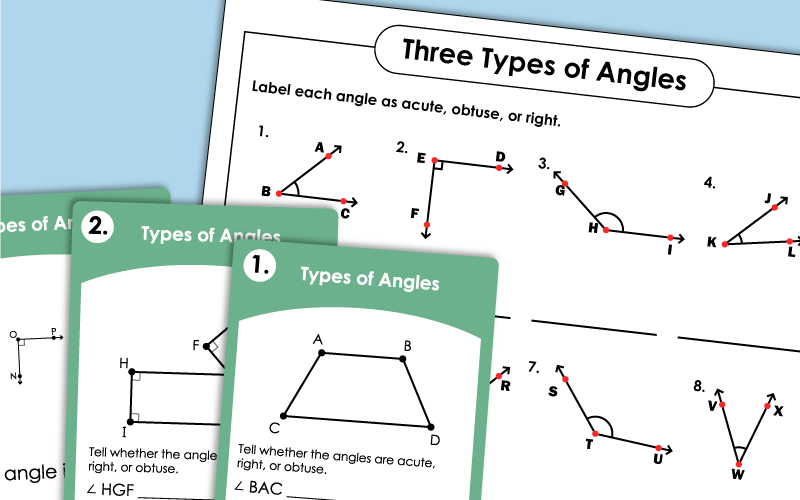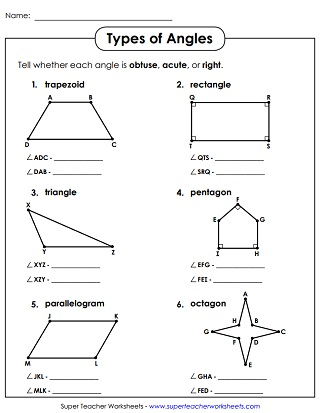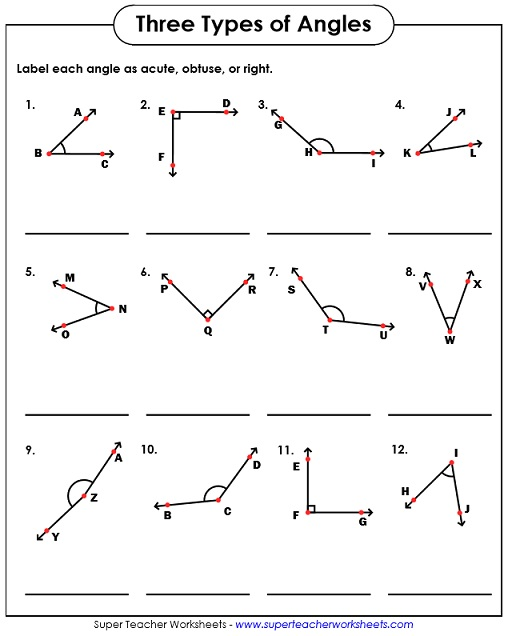# Types of Angles: Acute, Obtuse, Right

This page has printable geometry PDFs on angle types. Most worksheets require students to identify or analyze acute, obtuse, and right angles. The more advanced worksheets include straight and reflex angles too.Angle Types:
Actue, Obtuse, Right
Identify the right, acute and obtuse angles.
Tell whether each angle is acute, obtuse, or right. Angles are within polygons.
These printable task cards will challenge students to identify acute, right, and obtuse angles.
Look carefully at each illustration and find acute, obtuse, and right angles.
Estimate each angle's measurement, tell what type of angle it is, and write the angle name.
Two pages of notes with definitions of angles and types of angles.
Triangles:
Acute, Obtuse, Right
Identify the acute, obtuse, and right triangles.
Measuring and Drawing Angles

Practice measuring various angles with a protractor.

Angles: Complementary and Supplementary

On these activities, students will find complementary and supplementary angles by writing and solving simple equations.

More Geometry Worksheets

Our geometry section covers a wide variety of topics, including polygons, circles, solid shapes, area, perimeter, tessellations, congruent and similar shapes, congruency, and much more.

Sample Worksheet Images